### Group Representation of Global Intrinsic Symmetries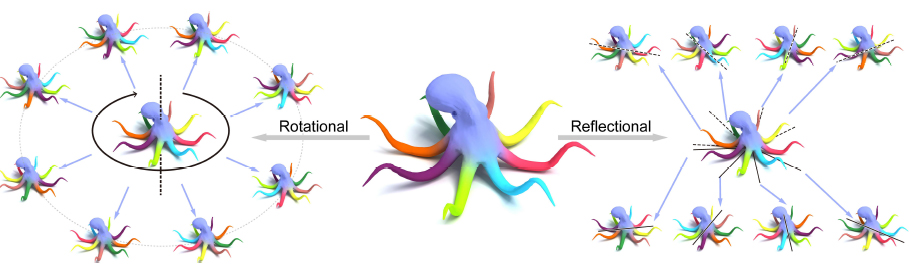Figure 1: Global intrinsic rotational (left) and reflectional (right) symmetries that we compute on an Octopus model, where symmetries are represented by the colors, comparing them with the original colored shape in the middle.

Abstract

Global intrinsic symmetry detection of 3D shapes has received considerable attentions in recent years. However, unlike extrinsic symmetry that can be represented compactly as a combination of an orthogonal matrix and a translation vector, representing the global intrinsic symmetry itself is still challenging. Most previous works based on point-to-point representations of global intrinsic symmetries can only find reflectional symmetries, and are inadequate for describing the structure of a global intrinsic symmetry group. In this paper, we propose a novel group representation of global intrinsic symmetries, which describes each global intrinsic symmetry as a linear transformation of functional space on shapes. If the eigenfunctions of the Laplace-Beltrami operator on shapes are chosen as the basis of functional space, the group representation has a block diagonal structure. We thus prove that the group representation of each symmetry can be uniquely determined from a small number of symmetric pairs of points under certain conditions, where the number of pairs is equal to the maximum multiplicity of eigenvalues of the Laplace-Beltrami operator. Based on solid theoretical analysis, we propose an efficient global intrinsic symmetry detection method, which is the first one able to detect all reflectional and rotational global intrinsic symmetries with a clear group structure description. Experimental results demonstrate the effectiveness of our approach.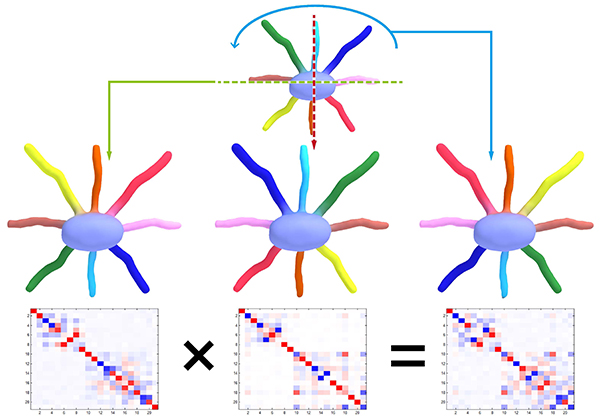Figure 2: The composition operation of global intrinsic symmetries is equal to a product of their 21x21 representation matrices. The middle row presents the symmetries via transferring colors from the top one onto the shape itself. Each matrix at bottom is the functional representation of the symmetry, as shown by the above.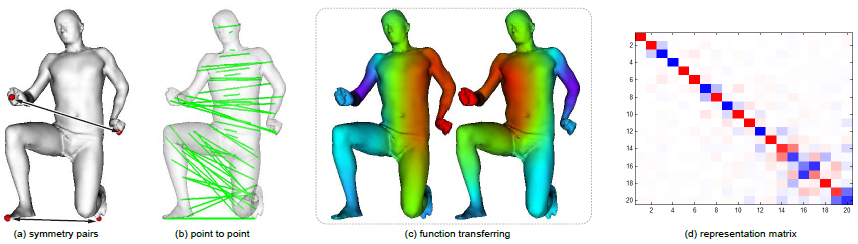Figure 3: Reflectional global intrinsic symmetry of a C2 shape is recovered from four symmetric pairs of points shown in (a), where (b)-(d) are different symmetry representations, that is, point-to-point correspondence (b), action of transferring colors from left to right (c), and the representation matrix (d), respectively.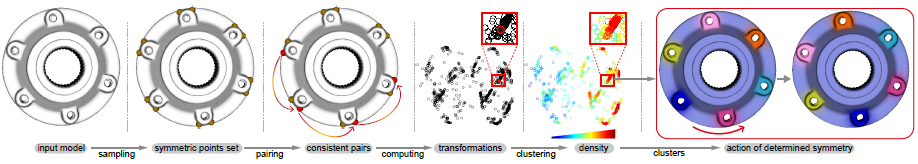Figure 4: The global intrinsic symmetry extraction pipeline of a screw model, where the maximum multiplicity of eigenvalues is m = 3. The sampling yields a symmetry-invariant set. Every three consistent pairs of points determine a transformed orthogonal matrix (2D embedding via PCA). Each cluster with higher density in the transformation space corresponds to a global intrinsic symmetry.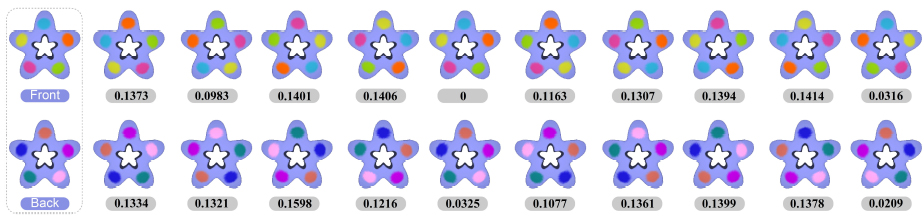Figure 5: Mean geodesic distance errors of the computed twenty global intrinsic symmetries (under each symmetry), which are much smaller than the tolerance error e = 3:92. The left column is the original color both in front and back view, while the other figures demonstrate symmetries by transferring the original color onto the shape itself in front view.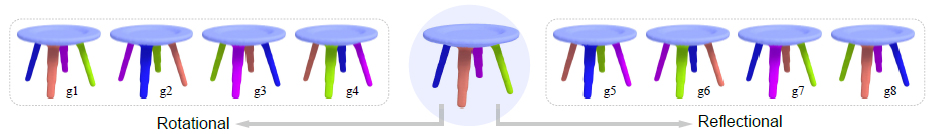Figure 6: The computed eight global intrinsic symmetries on a Table model, where each symmetry is demonstrated by transferring the original color (middle) onto the shape itself. The composition operation table of this global intrinsic symmetry group is show in Table 3.

[To reference our algorithm, code or data in a publication, please include the bibtex below and a link to this website.]

Acknowledgments

We thank the anonymous reviewers for their constructive comments. We also thank Maks Ovsjanikov for sharing the Octopus models used in Figures 1 and 3, and Alexander Bronstein et al. for sharing the codes of computing geodesic distances. This work was supported in part by NSFC (61402300, 61373160, 61522213, 61379090), 973 Program (2015CB352501), Guangdong Science and Technology Program (2014TX01X033, 2015A030312015, 2016A050503036), Shenzhen Innovation Program (JCYJ20151015151249564, 827-000196) and Excellent Young Scholar Fund of Shijiazhuang Tiedao University.

Bibtex

@article{Symmetry17,
title = {Group Representation of Global Intrinsic Symmetries},
author = {Hui Wang and Hui Huang},
journal = {Computer Graphics Forum (Proceedings of Pacific Graphics 2017)},
volume = {36},
issue = {7},
pages = {51–61},
year = {2017},
}